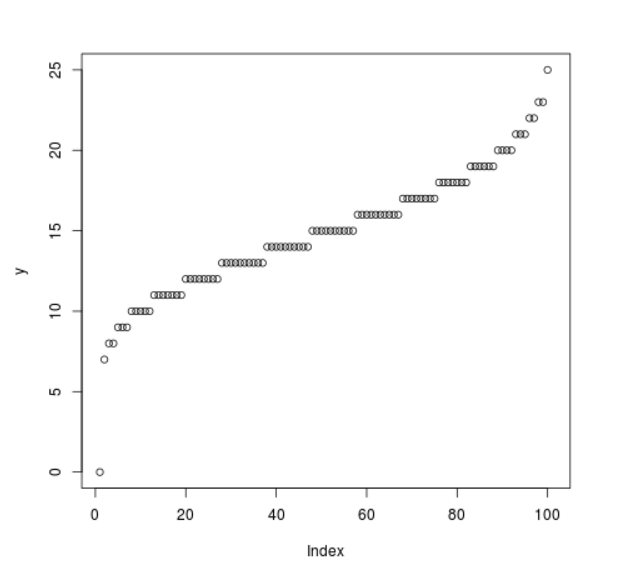# Compute the Value of Poisson Quantile Function in R Programming – qpois() Function

`qpois()` function in R Language is used to compute the value of Poisson Quantile Function. It creates a quantile density plot for poisson distribution.

Syntax: qpois(vec, lambda)

Parameters:
vec: Sequence of integer values
lambda: Average number of events per interval

Example 1:

 `# R program to compute  ` `# Poisson Quantile Density ` ` `  `# Sequence of x-values ` `x <``-` `seq(``0``, ``1``, by ``=` `.``01``) ` ` `  `# Calling qpois() Function ` `y <``-` `qpois(x, ``lambda` `=` `5``) ` `y `

Output:

```     0   1   1   1   1   2   2   2   2   2   2   2   2   3   3   3   3   3
   3   3   3   3   3   3   3   3   3   4   4   4   4   4   4   4   4   4
   4   4   4   4   4   4   4   4   4   5   5   5   5   5   5   5   5   5
   5   5   5   5   5   5   5   5   6   6   6   6   6   6   6   6   6   6
   6   6   6   6   6   7   7   7   7   7   7   7   7   7   7   8   8   8
   8   8   8   8   9   9   9  10  10  11 Inf
```

Example 2:

 `# R program to compute  ` `# Poisson Quantile Density ` ` `  `# Sequence of x-values ` `x <``-` `seq(``0``, ``1``, by ``=` `0.01``) ` ` `  `# Calling qpois() Function ` `y <``-` `qpois(x, ``lambda` `=` `15``) ` ` `  `# Plot a graph ` `plot(y) `

Output:Whether you're preparing for your first job interview or aiming to upskill in this ever-evolving tech landscape, GeeksforGeeks Courses are your key to success. We provide top-quality content at affordable prices, all geared towards accelerating your growth in a time-bound manner. Join the millions we've already empowered, and we're here to do the same for you. Don't miss out - check it out now!

Previous
Next